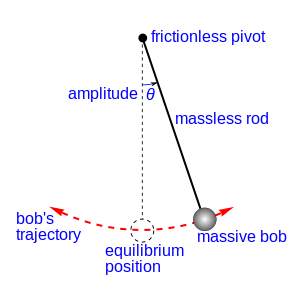## Simple Pendulum with Period, Length, Acceleration Calculator

 I want to calculate Period(T) Length(L) Acceleration of Gravity(g) Length(L) = m Acceleration of Gravity(g) = m/s2 Period(T) = s

A pendulum is a weight suspended from a pivot, when you give a force, it can swing freely.

The picture below shows a pendulum and it's variable. To "Simple gravity pendulum" it means no friction or air resistance.### Simple Pendulum formula

Period: T2=4PI2*L/g

where, g = Acceleration of Gravity = 9.8, L = Length, T = Period.

For example, when Length = 1, g = 9.8, The T = 2.84S.

Thinkcalculator.com provides you helpful and handy calculator resources.